Next: F.7 Window Correction (WC) Up: Calibration Files for Standard Previous: F.5 Electronic Position Correction

# F.6 Correction of Spatial Gain Variations (SGC)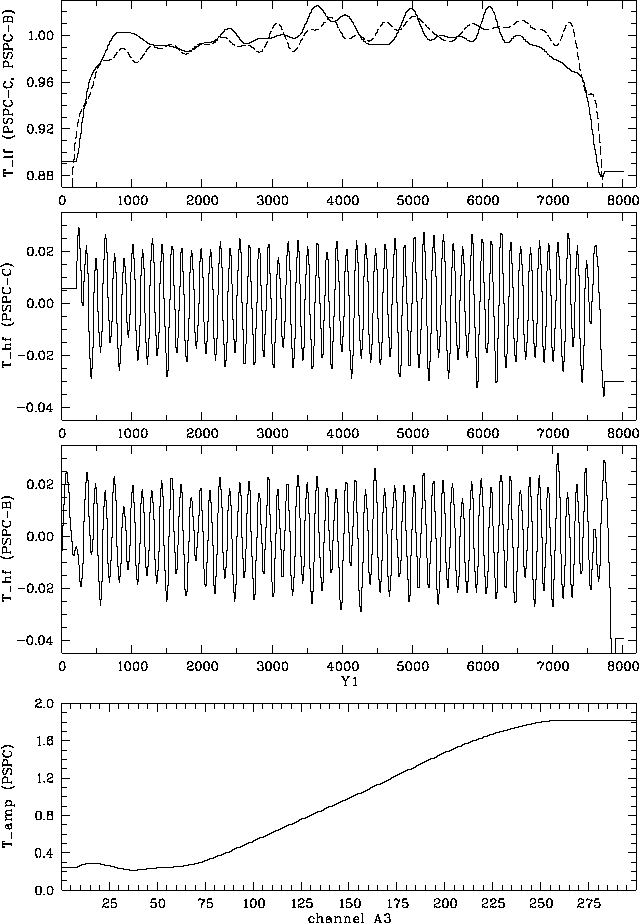Figure F.8: Spatial gain correction terms according to Eq.(F.12). Top panel: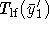for PSPC-C (solid) and PSPC-B (dashed). Middle panels: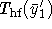for PSPC-C (upper) and PSPC-B (lower). Bottom panel: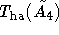(equal for both PSPCs).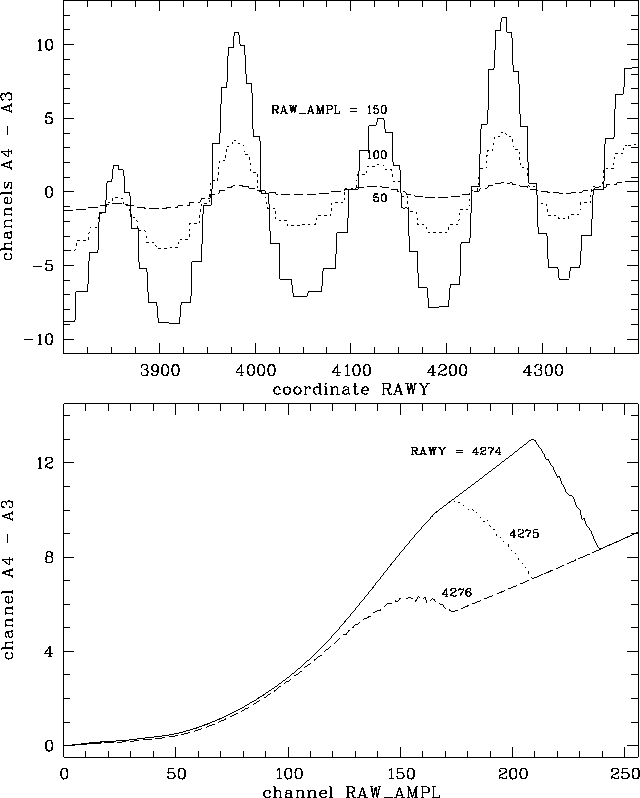Figure F.9: Effect of the spatial gain correction (SGC) in low-gain mode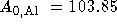for PSPC-B. Top: SGC as a function of the measured y-coordinate (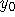, perpendicular to the anode wire direction) for 3 values of the measured pulse-height: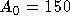(solid), 100 (dotted), and 50 (dashed). Bottom: SGC as a function of the measured pulse-height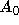for three different measured positions: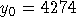(solid), 4275 (dotted), and 4276 (dashed). SGC does not depend on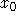(parallel to anode wire direction).,, andcorrespond in the figure to RAW_AMPL RAWX and RAWY, respectively.Figure F.10: Spatial gain correction effect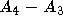as a function of PSPC-B position (, top to bottom) and raw amplitude (, left to right) for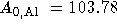; the color bar ranges from -22 to +22 channels.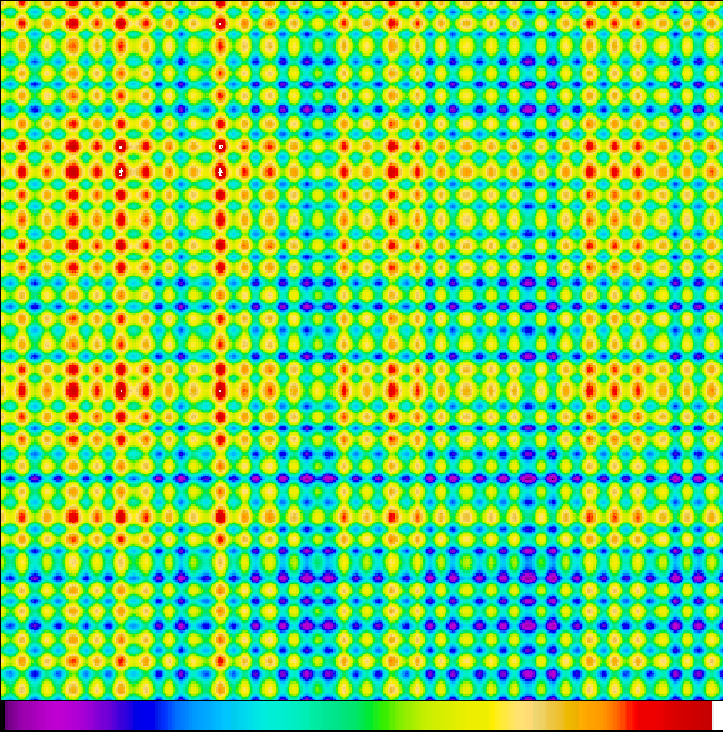Figure F.11: Spatial gain correction: effect of interchange of x and y axis as a function of PSPC-B position (detector coordinates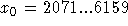and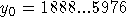, i.e., the central quarter of the PSPC): again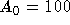andhave been used, the color bar ranges from -10 to +10 channels. The small-scale inhomogeneities reflect the anode wire spacing.

Besides the temporal gain variations (cf. Sect. F.4) spatial variations in the gain of the PSPC effect the measured pulse-height. Mechanical inaccuracies of the grid system lead to different electric field strengths and hence different gains. Additionally, the anode wire grid causes a ``binning'' with the period of the anode wire distance (1mm, about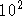detector pixels). During pre-flight ground calibrations using slit masks instead of the PSPC entrance window spatial gain variations have been determined. Significant effects have been found perpendicular to the anode wire direction (i.e., in y-direction) while parallel to the anodes the effect was negligible (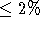). The effect also depends on the depth within the counter where the photon is absorbed (and therefore on the photon energy).

In SASS the pulse height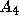is obtained depending on the electronic position corrected coordinate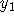according to Eq. (F.12). The energy-dependent term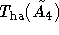has been determined at the Al K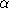line energy and should thus be normalized to unity at channel 151; it is shown in Fig. F.8 (bottom). The spatial corrections are expressed in a low-frequency term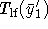(see Fig. F.8, top) and a high-frequency term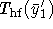(see Fig. F.8, middle) which reflects the anode wire spacing. In practice SASS uses(nearest integer of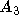); if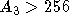then the value for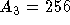is taken. The spatial correction tables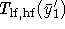used by SASS have 800 elements with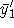; for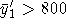the corresponding value foris used. This has no effect as the table values for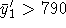(PSPC-B) and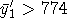(PSPC-C) are constant anyway.

Figure F.9 illustrates the effect of the spatial gain correction close to the detector center (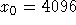, PSPC-B) in low-gain mode (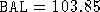). The top panel contains the relation of the effect with coordinate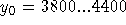(for 3 values of the measured pulse-height). The bottom panel shows how strong the effect depends on the position which results from the use of the roughly binned correction tables and introduces spectral artifacts. Figure F.10 gives the spatial gain correctionas a function of amplitude(from left to right, 1 to 256) and position(from top to bottom, 1 to 8192). Obviously, the effect becomes more important for higher channels. The color bar ranges from -22 to +22.

Another inconsistency is of a more severe nature. [Hasinger and Snowden1990] as well as Chap. 3 (PSPC) and Chap. 8 (SASS) use the x-coordinate as input in the correction formula Eq. (F.12) instead of the y-coordinate [Turner et al.1995]. This means, that no correction had been applied by SASS perpendicular to the anode wires as required but parallel to the anode wire direction. For positions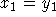this error vanishes. However, as the on-axis position is not in the detector center, (4119|3929) instead of (4096|4096), the target positions are significantly offset from this line and the improper applied correction has a net effect. On the other hand, this effect is reduced due to the wobble motion (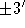, equivalent to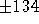detector pixels in x as well as in y direction) as the source position (relative to the detector) moves by more than the anode wire spacing. The wobble is performed parallel to x = y there is still a major effect on the amplitudes. This error has recently been corrected in SASS. The REV2 survey data as well as pointed data processed with SASS release 7.2 (or later) have the correct SGC applied. Figure F.11 gives the spatial gain correctionif the x and y axis are interchanged in the correction procedure (SASS versions 7.1 and lower) as a function of detector position (from left to right andfrom top to bottom). The color bar ranges here from -10 to +10.

After the spatial gain correction the amplitudeshould not depend on where the photon has been detected in the PSPC. Finally, the value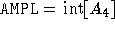is written into the photon event table (pulse-height invariant channel PI).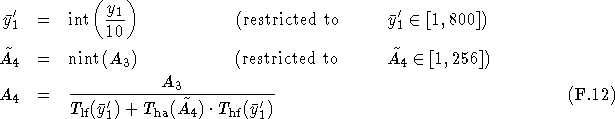Parameters:] pulse height of event, corrected for ADC non-linearity, gain saturation, and temporal variations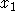detector x coordinate (electronic position correction applied)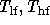position dependent correction tables (Fig. F.8. top and middle panels)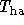energy dependent correction table (Fig. F.8, bottom panel)pulse height of event, corrected for ADC non-linearity, gain saturation, temporal and spatial variations (PI channel)
Remarks:y  direction are less than 2%, therefore corrections depending on y are not applied.
-
The correction tables depend on the detector used.

SASS source code: package PSPC, bubble CT, subroutine DCORG

SASS files: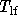and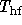for PSPC-C (Fig. F.8, top and middle panel)
p\$manpar:gain_kor3_b.datandfor PSPC-B\ (Fig. F.8, top and middle panel)
p\$manpar:gnampl_new.dat(Fig. F.8, bottom panel)Next: F.7 Window Correction (WC) Up: Calibration Files for Standard Previous: F.5 Electronic Position Correction

If you have problems/suggestions please send mail to rosat_svc@mpe-garching.mpg.de